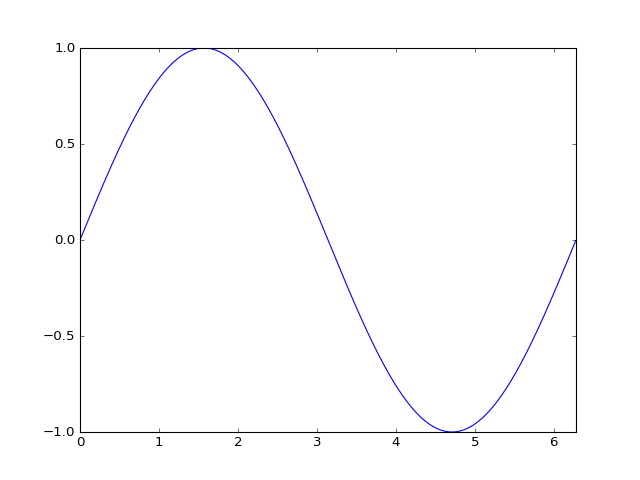# Animations¶

## Unit 17, Lecture 1¶

Numerical Methods and Statistics

#### Prof. Andrew White, April 5 2016¶

In :
%matplotlib notebook
import random
import numpy as np
import matplotlib.pyplot as plt
import scipy.stats
import matplotlib.animation as animation

In :
fig, ax = plt.subplots()

x = np.arange(0, 2*np.pi, 0.01)        # x-array
y = np.sin(x)
line, = ax.plot(x, y)
plt.xlim(0, 2*np.pi)
plt.show()In :
line.set_ydata(np.sin(x + np.pi))

In :
def move_line(dx=0.01, line=line):
line.set_ydata(np.sin(x + dx))
return fig

In :
#make an animation with 250 frames, where our phase shift goes from 0 to 2 pi
ani = animation.FuncAnimation(fig, move_line, np.linspace(0, 2 * np.pi, 250),blit=True)

In :
print(animation.writers.list())

['avconv', 'avconv_file']

In :
# Set up formatting for the movie files
Writer = animation.writers['avconv']
writer = Writer(fps=30, bitrate=1800, codec='h264')
ani.save('wave.mp4', writer=writer)


To get the link below, I just found where the resulting video file went. You may be able to just use "wave.mp4" if you're not using the jhub server.

In :
%%HTML
<video controls="" autoplay="" name="media" loop>
<source src="https://jhub.rochester.edu/user/[email protected]/files/local/wave.mp4" type="video/mp4">
</video>

In [ ]: